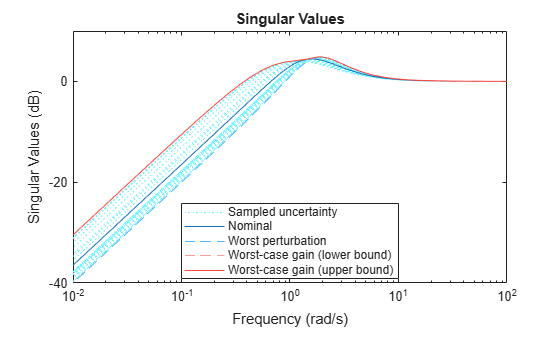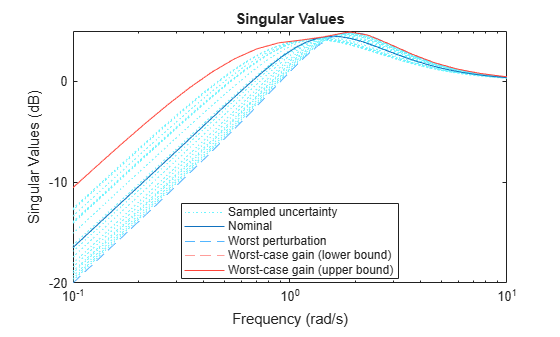Documentation

# wcsigma

Plot worst-case gain of uncertain system

## Syntax

``wcsigma(usys)``
``wcsigma(usys,w)``
``wcsigma(___,opts)``

## Description

example

````wcsigma(usys)` plots the nominal and worst-case gains of the uncertain system `usys` as a function of frequency. For multi-input, multi-output (MIMO) systems, gain refers to the largest singular value of the frequency response matrix. (See `sigma` for more information about singular values.) The plot includes:Nominal — Nominal gain of `usys`.Worst — The response falling within the uncertainty of `usys` that has the highest peak gain.Worst-case gain (lower bound) — The lowest possible worst-case gain at each frequency.Worst-case gain (upper bound) — The highest possible gain within the uncertainty at each frequency. This curve represents the envelope produced by finding the highest possible gain at each frequency.Sampled Uncertainty — 20 responses randomly sampled from `usys`.```

example

````wcsigma(usys,w)` focuses the plot on the frequencies specified by `w`.If `w` is a cell array of the form `{wmin,wmax}`, then `wcsigma` plots the worst-case gains in the range `{wmin,wmax}`.If `w` is an array of frequencies, then `wcsigma` plots the worst-case gains at each frequency in the array.```

example

````wcsigma(___,opts)` specifies additional options for the computation. Use `wcOptions` to create `opts`.```

## Examples

collapse all

Plot the worst-case gain of the following system:

`$sys=\frac{{s}^{2}+3s}{{s}^{2}+2s+a}.$`

The uncertain parameter a = 2 $±$ 1.

```a = ureal('a',2); usys = tf([1 3 0],[1 2 a]); wcsigma(usys)```The `Worst` curve identifies the single response within the uncertainty that yields the highest gain at any frequency. The `Worst-case gain (upper bound)` curve is the envelope produced by finding the highest gain within the uncertainty at each frequency.

The `Worst perturbation` curve identifies the combination of uncertain elements within the specified range that yields the highest overall gain. This perturbation corresponds to the `wcu` output of `wcgain`. The `Worst-case gain` curves show the lower and upper bounds on the worst-case gain at each frequency. For any perturbation within the specified uncertainty range, the principal gains (singular values) of the perturbed system lie below the `Worst-case gain (upper bound)` curve.

Focus the plot on the region between 0.1 and 10 rad/s.

```w = {0.1 10}; wcsigma(usys,w)```Examine the effect on the worst-case response of increasing the uncertainty range. To do this without changing the uncertainty specified in `usys`, use the `ULevel` option of `wcOptions`. This option scales the normalized uncertainty by the factor you specify. For example, examine the worst-case response a 50% greater uncertainty range.

```opts = wcOptions('ULevel',1.5); wcsigma(usys,w,opts)```The plot shows that increasing the uncertainty range substantially increases the worst-case gain at low frequencies.

## Input Arguments

collapse all

Dynamic system with uncertainty, specified as a `uss`, `ufrd`, `genss`, or `genfrd` model that contains uncertain elements.

For `genss` or `genfrd` models, `wcsigma` uses the current value of any tunable blocks and folds them into the known (not uncertain) part of the model.

Frequencies at which to plot worst-case gains, specified as the cell array `{wmin,wmax}` or as a vector of frequency values.

• If `w` is a cell array of the form `{wmin,wmax}`, then the function plots the worst-case gains at frequencies ranging between `wmin` and `wmax`.

• If `w` is a vector of frequencies, then the function plots the worst-case gains at each specified frequency. For example, use `logspace` to generate a row vector with logarithmically spaced frequency values.

Specify frequencies in units of rad/`TimeUnit`, where `TimeUnit` is the `TimeUnit` property of the model.

Options for worst-case computation, specified as an object you create with `wcOptions`. Setting certain options for `mussv` can improve the results of the worst-case calculation. See `wcOptions` for more information.

Example: `wcOptions('ULevel',2,'MussvOptions','m3')`

## Algorithms

`wcsigma` uses `wcgain` to compute the worst-case gains. Use the `opts` argument to set options for the `wcgain` algorithm.

`wcsigma` uses `usample` to compute the ```Sampled Uncertainty``` curves.